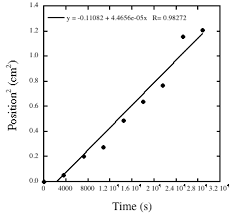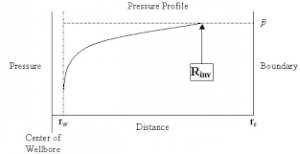## How to Calculate and Solve for Minimum Transient Time in Well Testing | The Calculator EncyclopediaThe image above represents minimum transient time.

To compute for the minimum transient time, five essential parameters are needed and these parameters are permeability (k), porosity (φ), reservoir radius (r), viscosity (μ) and total compressibility (CT).

The formula for calculating the minimum transient time:

t = 9.48 x 104 φ CT r² / k

Where;

t = Minimum Transient Time
k = Permeability
φ = Porosity
μ = Viscosity
CT = Total Compressibility

Let’s solve an example;
Given that the permeability is 11, porosity is 14, reservoir radius is 7, viscosity is 13 and total compressibility is 22.
Find the minimum transient time?

This implies that;

k = Permeability = 11
φ = Porosity = 14
r = Reservoir Radius = 7
μ = Viscosity = 13
CT = Total Compressibility = 22

t = 9.48 x 104 φ CT r² / k
t = 9.48 x 104 x 14 x 22 x 7² / 11
t = 9.48 x 104 x 14 x 22 x 49 / 11
t = 1430721600 / 11
t = 130065600

Therefore, the minimum transient time is 13006500.

## How to Calculate and Solve for Reservoir Radius and Well Spacing | The Calculator EncyclopediaThe image above represents the reservoir radius.

To compute for the reservoir radius, one essential parameter is needed and this parameter is Well Spacing (A).

The formula for calculating the reservoir radius:

re = √[43560A  / π]

Where;

A = Well Spacing

Let’s solve an example;
Given that the well spacing of an reservoir radius is 42. Find the reservoir radius?

This implies that;

A = Well Spacing = 42

re = √[43560A  / π]
re = √[43560 x 42 / π]
re = √[1829520 / π]
re = √582354.3
re = 763.12

Therefore, the reservoir radius is 763.12 ft.